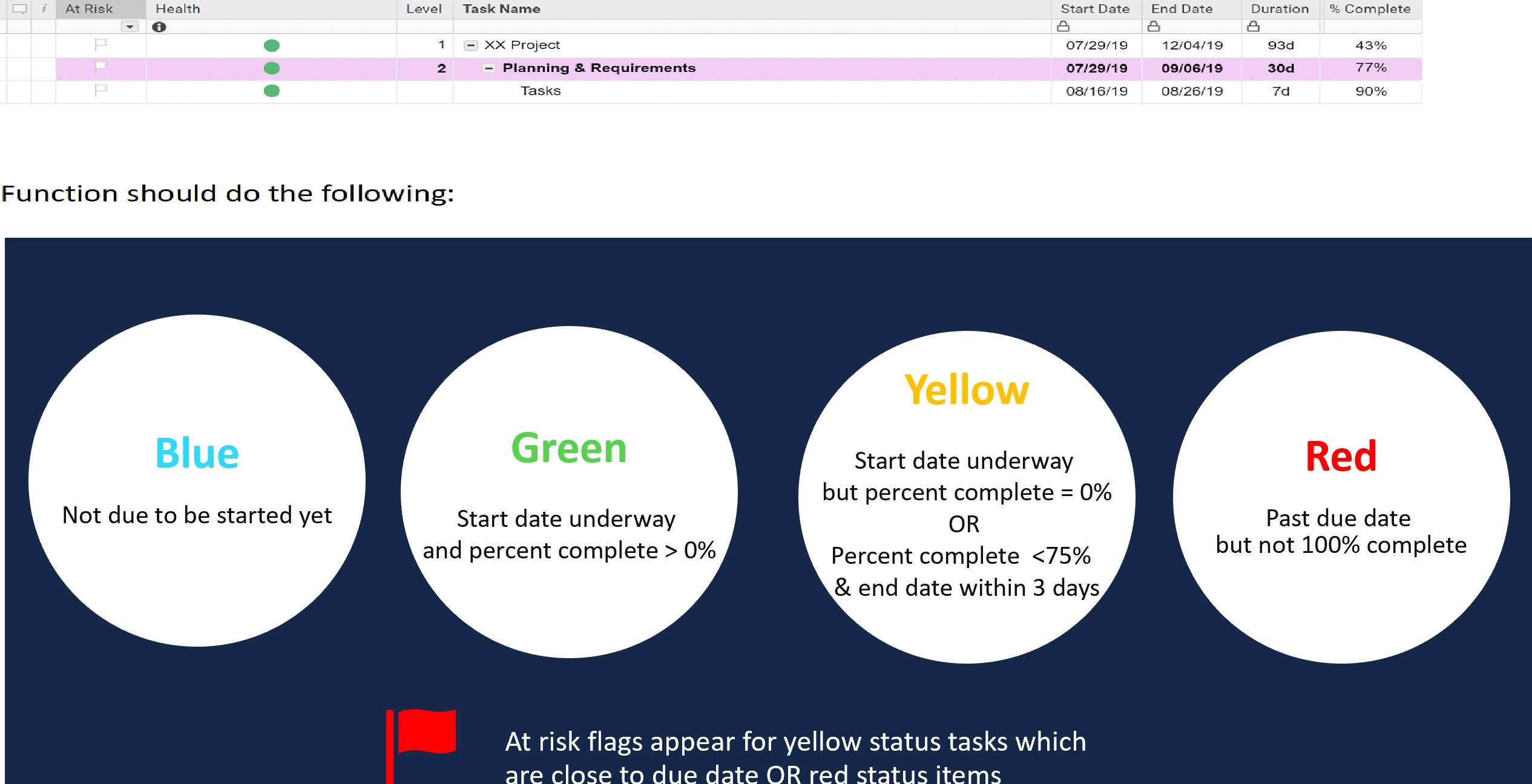# RGYB Automation

I'm having issues with the following formula and I'm not sure why.

=IF(ISBLANK([Start Date]@row), "Blue", IF([Start Date]@row >= TODAY(), "Blue", IF(AND([At Risk]@row = 1, [End Date]@row <= TODAY()), "Red", IF(AND([End Date]@row <= TODAY(3), [% Complete]@row < 0.75), "Yellow", IF(AND([Start Date]@row <= TODAY(), [% Complete]@row >= 0), "Green")))))

At Risk Formula below:  (for transparency)

=IF(COUNTIF(CHILDREN(), 1) > 0, 1, IF(AND([End Date]@row <= TODAY(3), [% Complete]@row < 0.75), 1, 0))

Problem Below:

Not sure why the health status isn't working once the end date <= TODAY(), "Red".  The formula works in almost all activities except for a few through our plan.  Maybe it's not structured correctly.  Any help would be much appreciated.